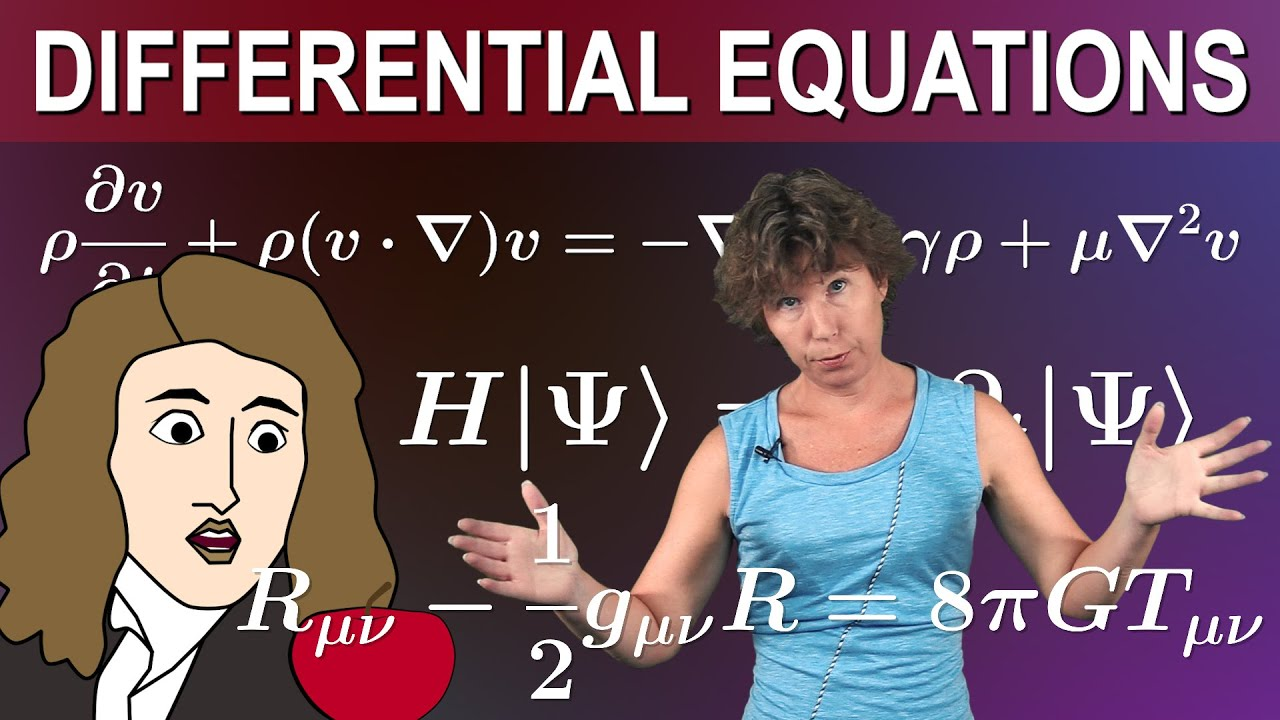# what is a differential equationMathematics

#### What are Differential Equations and how do they work?

Sabine Hossenfelder explains what differential equations are, go through two simple examples, explain the relevance of initial conditions and how differential equations generally work, and then discuss what this means to the question whether the future is determined already. Time Index: 0:00 Motivation and Content Summary 0:55 Example Disease Spread 3:25 Example Newton’s Law 5:18 […]